US4154508A - Rear conversion lens - Google Patents

Info

Publication number
US4154508A
US4154508A US05/773,796 US77379677A US4154508A US 4154508 A US4154508 A US 4154508A US 77379677 A US77379677 A US 77379677A US 4154508 A US4154508 A US 4154508A
Authority
US
United States
Prior art keywords
sub
lens
ν
represents
object side
Prior art date
Legal status (The legal status is an assumption and is not a legal conclusion. Google has not performed a legal analysis and makes no representation as to the accuracy of the status listed.)
Application number
US05/773,796
Inventor
Soichi Nakamura
Current Assignee (The listed assignees may be inaccurate. Google has not performed a legal analysis and makes no representation or warranty as to the accuracy of the list.)
Nikon Corp
Nippon Kogaku KK
Original Assignee
Nippon Kogaku KK
Priority date (The priority date is an assumption and is not a legal conclusion. Google has not performed a legal analysis and makes no representation as to the accuracy of the date listed.)
Filing date
Publication date
Priority to JP2557176A priority Critical patent/JPS5542725B2/ja
Priority to JP51-25571 priority
Application filed by Nippon Kogaku KK filed Critical Nippon Kogaku KK
Application granted granted Critical
Publication of US4154508A publication Critical patent/US4154508A/en
Assigned to NIKON CORPORATION, 2-3, MARUNOUCHI 3-CHOME, CHIYODA-KU, TOKYO, JAPAN reassignment NIKON CORPORATION, 2-3, MARUNOUCHI 3-CHOME, CHIYODA-KU, TOKYO, JAPAN CHANGE OF NAME (SEE DOCUMENT FOR DETAILS). EFFECTIVE APR. 1, 1988 Assignors: NIPPON KOGAKU, K.K.
Anticipated expiration legal-status Critical
Application status is Expired - Lifetime legal-status Critical

• 239000003570 air Substances 0 claims description 26
• 230000004075 alteration Effects 0 description 5
• 238000006243 chemical reaction Methods 0 abstract claims description title 69
• 239000002131 composite material Substances 0 claims description 6
• 238000010276 construction Methods 0 description 10
• 230000003247 decreasing Effects 0 description 1
• 230000001965 increased Effects 0 description 2
• 238000009740 moulding (composite fabrication) Methods 0 description 4
• 230000003287 optical Effects 0 description 3
• 230000002829 reduced Effects 0 description 2
• 230000001603 reducing Effects 0 description 1
• 230000011514 reflex Effects 0 description 1

Images

•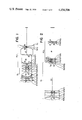•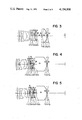•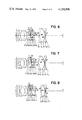•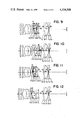•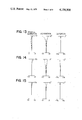•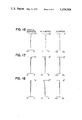•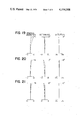•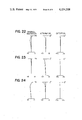•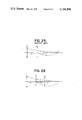Classifications

• GPHYSICS
• G02OPTICS
• G02BOPTICAL ELEMENTS, SYSTEMS, OR APPARATUS
• G02B9/00Optical objectives characterised both by the number of the components and their arrangements according to their sign, i.e. + or -
• G02B9/04Optical objectives characterised both by the number of the components and their arrangements according to their sign, i.e. + or - having two components only
• G02B9/10Optical objectives characterised both by the number of the components and their arrangements according to their sign, i.e. + or - having two components only one + and one - component
• GPHYSICS
• G02OPTICS
• G02BOPTICAL ELEMENTS, SYSTEMS, OR APPARATUS
• G02B15/00Optical objectives with means for varying the magnification
• G02B15/02Optical objectives with means for varying the magnification by changing, adding, or subtracting a part of the objective, e.g. convertible objective
• G02B15/10Optical objectives with means for varying the magnification by changing, adding, or subtracting a part of the objective, e.g. convertible objective by adding a part, e.g. close-up attachment

Abstract

A rear conversion lens system to be mounted on the image side of a photographic lens to enlarge the total focal length of the entire lens system including the photographic lens comprises a diverging group and a converging group disposed on the image side of the diverging group. The diverging group includes, as viewed in order from the object side, a first positive lens component, a negative lens component and a second positive lens component. The converging group includes, as viewed in order from the object side, a positive lens component and a negative lens component.

Description

BACKGROUND OF THE INVENTION

1. Field of the Invention

This invention relates to an attachment lens which may be mounted on the image side of a photographic lens to enlarge the focal length thereof, or a so-called rear conversion lens.

2. Description of the Prior Art

An attachment lens of this type which has heretofore been commercially available provides a negative lens system having a strong power and therefore, the Petzval sum thereof becomes negative, as a result of which the astigmatic difference between the sagittal plane and the meridional plane is particularly great, and accordingly, the other aberrations are also great. It may thus be said that a lens system having a conversion lens of such type mounted thereto to increase the focal length of the lens system is considerably inferior in performance to a fixed lens having a focal length equivalent thereto.

SUMMARY OF THE INVENTION

The present invention has, for its object, to provide a high performance rear conversion lens which comprises two lens groups, namely, a diverging lens group and a converging lens group, the diverging lens group basically consisting of three separate components, positive, negative and positive, and the converging lens group basically consisting of two separate components, positive and negative.

In accordance with a basic embodiment of the present invention, the rear conversion lens system to be mounted on the image side of a photographic lens to enlarge the total focal length of the entire lens system including the photographic lens comprises a diverging group and a converging group disposed on the image side of the diverging group, the diverging group including, as viewed in order from the object side, a first positive lens component, a negative lens component and a second positive lens component, the converging group including, as viewed in order from the object side, a positive lens component and a negative lens component.

The invention will become more fully apparent from the following detailed description thereof taken in conjunction with the accompanying drawings.

BRIEF DESCRIPTION OF THE DRAWINGS

FIGS. 1 to 12 are cross-sectional views of optical systems in which a standard lens is attached to the lens systems according to Embodiments 1 to 12 of the present invention.

FIGS. 13 to 24 illustrate various aberrations in the optical systems in which a standard lens is attached to the lens systems according to Embodiments 1 to 12 of the present invention.

FIG. 25 illustrates the principle of the usual rear converter.

FIG. 26 illustrates the principle of the present invention.

DESCRIPTION OF THE PREFERRED EMBODIMENTS

The principle of the usual rear conversion lens will first be described by reference to FIG. 25. In FIG. 25, let f be the focal length of the fixed focal length lens which provides a reference, fR the focal length of the rear conversion lens, d the distance between the principal planes of the two lenses, F the back focus of the composite lens system provided by the standard lens and the conversion lens, and b the distance from the back principal plane of the rear conversion lens to the back focus F, and assume that a=f-d. Then,

1/-a+1/b=1/fR                                              (1)

the lateral magnification β of the rear conversion lens is:

β=b/a

and the total focal length F is expressed as:

F=f·β

When the rear conversion lens in which β is constant is considered, a greatest possible value for a leads to a greater value b and as the result, the fR can be increased according to equation (1), so that the negative Petzval sum does not have to be increased. However, when various interchangeable lenses for a single lens reflex camera are considered, those lenses whose focal lengths are short are necessarily short in back focal length and the telephoto lenses are not so long in back focal length and thus, a is unavoidably small and accordingly, fR is also small, so that it is very difficult to correct the Petzval sum.

According to the present invention, this rear conversion fR is made into a two-group type to thereby decrease the Petzval sum in terms of power arrangement and the distance between the principal planes of the respective lens groups is decreased to increase the freedom of correction of the aberrations, thereby realizing a high performance rear conversion.

The principle of the rear conversion lens according to the present invention will hereinafter be described by reference to FIG. 26.

Let f1 and f2 be the focal lengths of the diverging lens group and the converging lens group respectively assuming that both lens groups are thin lenses, e the distance between the principal planes of the two groups, a the length as measured from the principal plane of the diverging lens group to the back focus of the standard lens, b the length as measured from the principal plane of the converging lens group to the final image point of the composite lens system, and r the length as measured from the principal plane of the diverging lens group to the image point provided by the diverging lens group. Then,

1/-a+1/r=1/f.sub.1                                         (2)

1/(-r+e)+1/b=1/f.sub.2                                     (3)

Assuming that the focal length of the entire rear conversion lens is fR,

1/fR=1/f.sub.1 +1/f.sub.2 -e/f.sub.1 f.sub.2

Also, the magnification β of the entire rear conversion lens is expressed as:

β={r/a}·{b/(r-e)}                            (4)

If the diverging and the converging lens groups of the rear conversion lens respectively comprise k single lenses and h single lenses, ##EQU1## where ND and NC represent the equivalent refractive indices of the respective groups when they are each regarded as a single lens, qi and qj represent the focal lengths of the respective components, and ni and nj represent the refractive indices of the respective components.

Assuming that the Petzval sum of the entire rear conversion lens is P,

p=1/f.sub.1 N.sub.D +1/f.sub.C N.sub.2                     (5)

by equations (2), (3), (4) and (5), the following is given: ##EQU2## Also, from equation (2),

f.sub.1 =ra/(a-r)                                          (7)

From equation (4),

e=r-br/βa                                             (8)

From equations (3) and (4),

f.sub.2 =rb/(r-aβ)                                    (9)

Therefore, if a, b, P, ND, NC and β and given, r can be determined by equation (6) and f1, f2 and e can be determined by equations (7), (8) and (9). Thus, the skeleton of such optical system, namely, f1, f2 and e, are determined by the value of the Petzval sum P required for such type of rear conversion lens, how the equivalent refractive index ND of the diverging group can be made and how low the equivalent index NC of the converging group can be made without involving aggravated aberrations.

By realizing the following relations;

1.5<ND <2.9 and

0.6<NC <1.3,

a rear conversion lens in accordance with the invention is attained which has very well balanced aberrations.

Further, the fact that the present lens system is of the two-group type requires achromatization of the forward i.e. diverging and the rearward i.e. converging group to be accomplished, and assuming that the refractive index and Abbe number of the positive lens component forming the forward group are nDP and νVP, respectively, and that those of the positive lens component forming the forward group and nDN and νDN, respectively, they must be in the following relations:

nDP<nDN and

νDP<νDN.

Assuming that the refractive index and the Abbe number of the positive lens component forming the rear group are nCP and νCP, respectively, and that those of the negative lens component forming the rear group are nCN and νCN, respectively, they must be in the following relations:

nCP<nCN and

νCP<νCN.

Some embodiments of the present invention will now be described in detail.

FIG. 1 shows the lens arrangement when the rear conversion lens RCL according to a first embodiment of the present invention is mounted to a standard lens SL having a focal length f=51.6 and a F-number 2.0.

Embodiment 1 is a basic one of the present invention, in which the forward group comprises three components, positive, negative and positive, and

N.sub.D =1.75,

while the rearward group comprises two positive and negative components and NC =0.921 is realized, thereby enabling the provision of a rear conversion lens in which the Petzval sum is -0.0061.

______________________________________f.sub.1 = -50.48869,               f.sub.2 = 208.1101,e = 20.25835,       fR = -76.492.______________________________________

FIG. 2 shows the lens arrangement when the rear conversion lens RCL according to a second embodiment of the present invention is mounted to a standard lens SL having a focal length f=300 mm and a F-number 4.5.

Embodiment 2 has the following numerical values:

______________________________________f.sub.1 = -96.8024, f.sub.2 = 281.131e = 69.217,N.sub.D = 1.906     N.sub.C = 1.1292,fR = -236.415       p = -0.00227.______________________________________

In Embodiment (3) shown in FIG. 3, which is substantially similar in construction to Embodiment 1, the positive component of the rearward group is provided by a negative and a positive element cemented together, thereby intending to improve the Petzval sum. In this embodiment,

______________________________________N.sub.D = 1.664,    N.sub.C = 0.7664,P = -0.00563.______________________________________

In Embodiment 4 shown in FIG. 4, which is again substantially similar in construction to Embodiment 1, the second positive component of the forward group is divided into two components, positive and negative, so that the forward group is provided by four components, positive, negative, positive and negative. By this, ND =2.311 and NC =0.953 can be realized and the Petzval sum P can be reduced to P=0.0035.

In Embodiment 5 shown in FIG. 5, which is also substantially similar in construction to Embodiment 1, the first positive component of the forward group is divided into two components, negative and positive, so that the forward group is provided by four components, negative, positive, negative and positive. By this, ND =2.23 and NC =0.965 can be realized and the Petzval sum P can be reduced to P=-0.00389.

In Embodiment 6 shown in FIG. 6, which is substantially similar in construction to Embodiment 4, the positive component of the rearward group is provided by a negative and a positive component cemented together to thereby further reduce the Petzval sum. In this embodiment,

______________________________________N.sub.D = 2.295,    N.sub.C = 0.7183,P = -0.00194.______________________________________

In embodiment 7 shown in FIG. 7, which is substantially similar in construction to Embodiment 6, the first positive and the first negative component of the forward group are cemented together and

______________________________________N.sub.D = 2.179,    N.sub.C = 0.679,P = -0.00201.______________________________________

In Embodiment 8 shown in FIG. 8, which is substantially similar in construction to Embodiment 6, the second positive and the second negative component of the forward group are cemented together, thereby succeeding in realizing:

______________________________________N.sub.D = 2.7395,   N.sub.C = 0.7555,P = -0.0009.______________________________________

In Embodiment 9 shown in FIG. 9, which is substantially similar in construction to Embodiment 5, the positive component of the rearward group is provided by a negative and a positive component cemented together, thereby reducing the Petzval sum. In this embodiment,

______________________________________N.sub.D = 2.3058    N.sub.C = 0.7676P = -0.00232.______________________________________

In Embodiment 10 shown in FIG. 10, which is substantially similar in construction to Embodiment 9, the first positive and the second negative component of the forward group are cemented together, thereby realizing:

______________________________________N.sub.D = 2.16,     N.sub.C = 0.7652,p = -0.00289.______________________________________

In Embodiment 11 shown in FIG. 11, which is substantially similar in construction to Embodiment 9, the second negative and the second positive component of the forward group are cemented together, thereby realizing:

______________________________________N.sub.D = 2.369,    N.sub.C = 0.7701,P = -0.00211.______________________________________

In Embodiment 12 shown in FIG. 12, which is again similar in construction to Embodiment 9, the first positive, the second negative and the second positive component of the first group are cemented together, thereby realizing:

______________________________________N.sub.D = 2.427,    N.sub.C = 0.8214,P = -0.00231.______________________________________

Now, the numerical data of the various embodiments of the present invention will be shown below, but before that, examples will first be shown of the typical Gauss type standard lens to which is to be mounted the rear conversion lens of the present invention used in any of Embodiment 1 and Embodiments 3 to 12, and of the typical telephoto lens to which is to be mounted the rear conversion lens used in Embodiment 2.

In the tables below, R1, R2 and so on represent the radii of the successive lens surfaces as viewed from the object side, D1, D2 and so on represent the thickness of, or the distances between, the successive lens surfaces as viewed from the object side, and N1, N2 and so on and ν1, ν2 and so on represent the refractive indices and the Abbe numbers, respectively, of the successive lenses.

The standard lens used in any of Embodiment 1 and Embodiments 3 to 12 has the following data:

______________________________________f = 51.6   F2         Angle of view 2 ω = 46°______________________________________R.sub.1 = 36.011      D.sub.1 = 3.41                 N.sub.1 = 1.62299                             V.sub.1 = 58.1R.sub.2 = 114.87      D.sub.2 0.11R.sub.3 = 22.46      D.sub.3 = 4.55                 N.sub.2 1.6779                             V.sub.2 = 55.5R.sub.4 = 81.84      D.sub.4 = 1.84R.sub.5 = 102.18      D.sub.5 = 1.98                 N.sub.3 = 1.62588                             V.sub.3 = 35.6R.sub.6 = 14.954      D.sub.6 = 9.4R.sub.7 = -16.1      D.sub.7 = 1.18                 N.sub.4 = 1.62606                             V.sub.4 = 39.1R.sub.8 = 69.04      D.sub.8 = 5.91                 N.sub.5 = 1.63854                             V.sub.5 = 55.5R.sub.9 = -21.13      D.sub.9 = 0.11R.sub.10 = 114.52      D.sub.10  = 3.33                 N.sub.6 1.63854                             V.sub.6 = 55.5R.sub.11 = -43.546______________________________________

The standard lens used in Embodiment 2:

______________________________________f = 300    F4.5       Angle of view 2 ω = 8° 10.sup.f______________________________________R.sub.1 = 106.0      D.sub.1 = 11.0                 N.sub.1 = 1.48606                             V.sub.1 = 81.9R.sub.2 = -151.0      D.sub.2 = 4.0R.sub.3 = -144.0      D.sub.3 = 3.0                 N.sub.2 = 1.744                             V.sub.2 = 44.9R.sub.4 = 130.0      D.sub.4 12.0                 N.sub.3 = 1.6393                             V.sub.3 = 45.0R.sub.5 = 650.0      D.sub.5 1.0R.sub.6 = 95.0      D.sub.6 = 7.7                 N.sub.4 = 1.48606                             V.sub.4 = 81.9R.sub.7 878.8      D.sub.7 = 119.3R.sub.8 = -43.0      D.sub.8 = 1.0                 N.sub.5 = 1.62041                             V.sub.5 = 60.3R.sub.9 = 150.0      D.sub.9 = 3.5                 N.sub.6 = 1.62004                             V.sub.6 = 36.3R.sub.10 = -104.82______________________________________

Examples of the rear conversion lens will be shown below. The total focal length of the rear conversion lens when mounted to the above-shown standard lens is f=103.2 F4 and 2.sup.ω =24.4° in any of Embodiment 1 and Embodiments 3 to 12, and is f=600, F9 and 2.sup.ω= 4°10', that is, the magnification of the rear conversion is β=2 in all of the embodiments.

In the tables below, r represents the curvature radius of each lens surface, d represents the center thickness of each lens or the air space between adjacent ones of the lenses, excepting d0 which represents the air space between the rear conversion lens and the standard lens, n represents the refractive index of each lens, ν represents the Abbe number of each lens, and the subscript numbers represent the order from the object side.

EXAMPLE 1

______________________________________                d.sub.0 = 0.7613     r.sub.1 = 84.84                d.sub.1 = 3.0                         n.sub.1 = 1.59507                                  ν.sub.1 = 35.6     r.sub.2 = -67.022                d.sub.2 = 2.3     r.sub.3 = -65.251                d.sub.3 = 1.0                         n.sub.2 = 1.74443                                  ν.sub.2 = 49.4f.sub.1   r.sub.4 = 16.493                d.sub.4 = 1.4     r.sub.5 = 35.215                d.sub.5 = 18.9                         n.sub.3 = 1.59507                                  ν.sub.3 = 35.6     r.sub.6 = -658.806                d.sub.6 = 0.514     r.sub.7 = 877.266                d.sub.7 = 1.2                         n.sub.4 = 1.50137                                  ν.sub.4 = 56.5     r.sub.8 = -21.399                d.sub.8 = 0.1f.sub.2   r.sub.9 = -332.183                d.sub.9 =  1.0                         n.sub.5 = 1.80454                                  ν.sub.5 = 39.5     r.sub.10 = 47.332______________________________________
EXAMPLE 2

______________________________________                d.sub.0 = 1.5423     r.sub.1 = 179.709                d.sub.1 = 3.7                         n.sub.1 = 1.59507                                  ν.sub.1 = 35.6     r.sub.2 = -90.827                d.sub.2 = 2.0     r.sub.3 =-109.097                d.sub.3 = 2.1                         n.sub.2 = 1.74443                                  ν.sub.2 = 49.4f.sub.1   r.sub.4 = 32.52                d.sub.4 = 2.1     r.sub.5 = 40.56                d.sub.5 = 4.2                         n.sub.3 = 1.59507                                  ν.sub.3 = 35.6     r.sub.6 = 99.049                d.sub.6 = 66.24     r.sub.7 = 317.64                d.sub.7 = 6.2                         n.sub.4 = 1.50137                                  ν.sub.4 = 56.5     r.sub.8 = -55.266                d.sub.8 = 0.2f.sub.2   r.sub.9 = -195.754                d.sub.9 = 1.8                         n.sub.5 = 1.80454                                  ν.sub.5 = 39.5     r.sub.10 = 267.716______________________________________
EXAMPLE 3

______________________________________                d.sub.0 = 0.52644     r.sub.1 = 187.587                d.sub.1 = 3.0                         n.sub.1 = 1.59507                                  ν.sub.1 = 35.6     r.sub.2 = -47.144                d.sub.2 = 3.3     r.sub.3 = -35.794                d.sub.3 = 1.0                         n.sub.2 = 1.74443                                  ν.sub.2 = 49.4f.sub.1   r.sub.4 = 19.353                d.sub.4 = 2.3     r.sub.5 = 44.11                d.sub.5 = 3.4                         n.sub.3 = 1.59507                                  ν.sub.3 = 35.6     r.sub.6 = -110.232                d.sub.6 = 11.275     r.sub.7 = 114.19                d.sub.7 = 2.0                         n.sub.4 = 1.6968                                  ν.sub.4 = 55.6     r.sub.8 = 34.505                d.sub.8 = 11.1                         n.sub.5 = 1.50137                                  ν.sub.5 = 56.5f.sub.2     r.sub.9 = -21.903                d.sub.9 = 0.1     r.sub.10 = -92.338                d.sub.10 = 1.4                         n.sub.6 = 1.80454                                  ν.sub.6 = 39.5     r.sub.11 = 80.765______________________________________
EXAMPLE 4

______________________________________                 d.sub.0 = 0.8448     r.sub.1 = 7602.182                 d.sub.1 = 2.5                         n.sub.1 = 1.59507                                  ν.sub.1 = 35.6     r.sub.2 = -85.234                 d.sub.2 = 0.1     r.sub.3 = 111.37                 d.sub.3 = 1.0                         n.sub.2 = 1.74443                                  ν.sub.2 = 49.4     r.sub.4 = 16.963                 d.sub.4 = 1.0f.sub.1   r.sub.5 = 27.323                 d.sub.5 = 4.9                         n.sub.3 = 1.59507                                  ν.sub.3 = 35.6     r.sub.6 = -27.976                 d.sub.6 = 1.1     r.sub.7 = -25.116                 d.sub.7 = 1.0                         n.sub.4 = 1.79668                                  ν.sub.4 = 45.4     r.sub.8 = 439.936                 d.sub.8 = 2.645     r.sub.9 = 1743.856                 d.sub.9 = 28.23                         n.sub.5 =  1.50137                                  ν.sub.5 = 56.5     r.sub.10 = -22.944                 d.sub.10 = 0.1f.sub.2   r.sub.11  = -294.545                 d.sub.11 = 1.0                         n.sub.6 = 1.80454                                  ν.sub.6 = 39.5     r.sub.12 = 54.449______________________________________
EXAMPLE 5

______________________________________                d.sub.0 = 0.9271     r.sub.1 = 36.295                d.sub.1 = 1.0                         n.sub.1 = 1.79668                                  ν.sub.1 = 45.0     r.sub.2 = 17.706                d.sub.2 = 1.2     r.sub.3 = 32.624                d.sub.3 = 4.7                         n.sub.2 = 1.59507                                  ν.sub.2 = 35.6     r.sub.4 = -28.183                d.sub.4 = 1.2f.sub.1   r.sub.5 = -27.231                d.sub.5 = 1.0                         n.sub.3 = 1.77279                                  ν.sub.3 = 49.4     r.sub.6 = 55.411                d.sub.6 = 1.0     r.sub.7 = 58.841                d.sub.7 = 1.55                         n.sub.4 = 1.59507                                  ν.sub.4 = 35.6     r.sub.8 = 117.020                d.sub.8 = 4.12     r.sub.9 = 1086.312                d.sub.9 = 25.3                         n.sub.5 = 1.50137                                  ν.sub.5 = 56.5     r.sub.10 = -24.049                d.sub.10 = 0.1f.sub.2   r.sub.11 = -185.481                d.sub.11 = 1.0                         n.sub.6 = 1.80454                                  ν.sub.6 = 39.5     r.sub.12 = 65.497______________________________________
EXAMPLE 6

______________________________________                 d.sub.0 =0.96135     r.sub.1 =∞                 d.sub.1 =2.5                          n.sub.1 =1.59507                                   ν.sub.1 =35.6     r.sub.2 =-81.319                 d.sub.2 =0.1     r.sub.3 =401.867                 d.sub.3 =1.0                          n.sub.2 =1.74443                                   ν.sub.2 =49.4     r.sub.4 =18.181                 d.sub.4 =1.4f.sub.1   r.sub.5 =28.13                 d.sub.5 =5.3                          n.sub.3 =1.59507                                   ν.sub.3 =35.6     r.sub.6 =-24.535                 d.sub.6 =1.5     r.sub.7 =-21.934                 d.sub.7 =1.0                          n.sub.4 =1.79668                                   ν.sub.4 =45.4     r.sub.8 =-472.423                 d.sub.8 =11.728     r.sub.9 =88.894                 d.sub.9 =1.5                          n.sub.5 =1.79877                                   ν.sub.5 =44.3     r.sub.10 =33.579                 d.sub.10 =12.6                          n.sub.6 =1.50137                                   ν.sub.6 =56.5f.sub.2     r.sub.11 =-22.556                 d.sub.11 =0.1     r.sub. 12 =-152.552                 d.sub.12 =1.0                          n.sub.7 =1.80454                                   ν.sub.7 =39.5     r.sub.13 =64.571______________________________________
EXAMPLE 7

______________________________________                 d.sub.0 =1.954     r.sub.1 =87.4                 d.sub.1 =2.5                          n.sub.1 =1.59507                                   ν.sub.1 =35.6     r.sub.2 =576.216                 d.sub.2 =1.0                          n.sub.2 =1.74443                                   ν.sub.2 =49.4     r.sub.3 =17.692                 d.sub.3 =1.4f.sub.1     r.sub.4 =36.939                 d.sub.4 =5.2                          n.sub.3 =1.59507                                   ν.sub.3 =35.6     r.sub.5 =-20.532                 d.sub.5 =2.0     r.sub.6 =-19.333                 d.sub.6 =1.0                          n.sub.4 =1.79877                                   ν.sub.4 =44.3     r.sub.7 =-79.971                 d.sub.7 =8.469     r.sub.8 =86.861                 d.sub.8 =1.5                          n.sub.5 =1.79877                                   ν.sub.5 =44.3     r.sub.9 =30.221                 d.sub.9 =13.9                          n.sub.6 =1.50137                                   ν.sub.6 =56.5f.sub.2     r.sub.10 =-20.432                 d.sub.10 =0.1     r.sub.11 =-188.213                 d.sub.11 =1.0                          n.sub.7 =1.80454                                   ν.sub.7 =39.5     r.sub.12 =53.325______________________________________
EXAMPLE 8

______________________________________                 d.sub.0 =1.2278     r.sub.1 =-167.947                 d.sub.1 =2.5                           n.sub.1 =1.59507                                   ν.sub.1 =35.6     r.sub.2 =-51.604                 d.sub.2 =0.1     r.sub.3 =97.027                 d.sub.3 =1.0                           n.sub.2 =1.74443                                   ν.sub.2 =49.4f.sub.1     r.sub.4 =16.21                 d.sub.4 =1.8     r.sub.5 =23.202                 d.sub.5 =7.9                           n.sub.3 =1.59507                                   ν.sub.3 =35.6     r.sub.6 =-17.735                 d.sub.6 =1.0                           n.sub.4 =1.79631                                   ν.sub.4 =40.8     r.sub.7 =182.618                 d.sub.7 =13.16     r.sub.8 =104.359                 d.sub.8 =1.5                           n.sub.5 =1.7335                                   ν.sub.5 =51.0     r.sub.9 =34.774                 d.sub.9 =9.2                           n.sub.6 =1.50137                                   ν.sub.6 =56.5f.sub.2     r.sub.10 =-22.416                 d.sub.10 =0.1     r.sub.11 =-160.252                 d.sub.11 =1.0                           n.sub.7 =1.80454                                   ν.sub.7 = 39.5     r.sub.12 =61.571______________________________________
EXAMPLE 9

______________________________________                 d.sub.0 =1.02869     r.sub.1 =37.224                 d.sub.1 =1.0                           n.sub.1 =1.79668                                   ν.sub.1 =45.4     r.sub.2 =17.855                 d.sub.2 =1.15     r.sub.3 =31.0                 d.sub.3 =4.8                           n.sub.2 =1.59507                                   ν.sub.2 =35.6f.sub.1     r.sub.4 =-27.118                 d.sub.4 =1.1     r.sub.5 =-26.049                 d.sub.5 =1.0                           n.sub.3 =1.77279                                   ν.sub.3 =49.4     r.sub.6 =41.192                 d.sub.6 =1.0     r.sub.7 =45.961                 d.sub.7 =2.6                           n.sub.4 =1.59507                                   ν.sub.4 =35.6     r.sub.8 =165.452                 d.sub.8 =13.1     r.sub.9 =98.846                 d.sub.9 =1.5                           n.sub.5 =1.79631                                   ν.sub.5 =40.8     r.sub.10 =36.72                 d.sub.10 =10.0                           n.sub.6 =1.51823                                   ν.sub.6 =59.0f.sub.2     r.sub.11 =-23.342                 d.sub.11 =0.1     r.sub.12 =-91.709                 d.sub.12 =1.0                           n.sub.7 =1.78797                                   ν.sub.7 =47.5     r.sub.13 =83.740______________________________________
EXAMPLE 10

______________________________________                 d.sub.0 =3.093     r.sub.1 =24.242                 d.sub.1 =1.0                          n.sub.1 =1.744                                   ν.sub.1 =44.9     r.sub.2 =14.539                 d.sub.2 =7.36     r.sub.3 =40.75                 d.sub.3 =2.7                          n.sub.2 =1.59507                                   ν.sub.2 =35.6f.sub.1     r.sub.4 =-73.12                 d.sub.4 =1.0                          n.sub.3 =1.77279                                   ν.sub.3 =49.4     r.sub.5 =25.676                 d.sub.5 =0.1     r.sub.6 =26.376                 d.sub.6 =5.1                          n.sub.4 =1.59507                                   ν.sub.4 =35.6     r.sub.7 =129.070                 d.sub.7 =6.25     r.sub.8 =116.7                 d.sub.8 =1.5                          n.sub.5 =1.79631                                   ν.sub.5 =40.8     r.sub.9 =41.2                 d.sub.9 =11.0                          n.sub.6 =1.51823                                   ν.sub.6 =59.0f.sub.2     r.sub.10 =-21.21                 d.sub.10 =0.1     r.sub.11 =-152.348                 d.sub.11 =1.0                          n.sub.7 =1.78797                                   ν.sub.7 =47.5     r.sub.12 =53.434______________________________________
EXAMPLE 11

______________________________________                 d.sub.0 =0.6917     r.sub.1 =37.545                 d.sub.1 =1.0                           n.sub.1 =1.79668                                   ν.sub.1 =45.4     r.sub.2 =17.681                 d.sub.2 =1.3     r.sub.3 =33.0                 d.sub.3 =4.8                           n.sub.2 =1.59507                                   ν.sub.2 =35.6f.sub.1     r.sub.4 =-26.174                 d.sub.4 =1.0     r.sub.5 =-25.59                 d.sub.5 =1.0                           n.sub.3 =1.77279                                   ν.sub.3 =49.4     r.sub.6 =40.631                 d.sub.6 =2.75                           n.sub.4 =1.59507                                   ν.sub.4 =35.6     r.sub.7 =136.497                 d.sub.7 =14.29     r.sub.8 =100.545                 d.sub.8 =1.5                           n.sub.5 =1.79631                                   ν.sub.5 =40.8     r.sub.9 =37.535                 d.sub.9 =10.0                           n.sub.6 = 1.51823                                   ν.sub.6 =59.0f.sub.2     r.sub.10 =-23.269                 d.sub.10 =0.1     r.sub.11 =-87.1                 d.sub.11 =1.0                           n.sub.7 =1.78797                                   ν .sub.7 =47.5     r.sub.12 =87.1______________________________________
EXAMPLE 12

______________________________________                  d.sub.0 =0.9054     r.sub.1 =29.797                  d.sub.1 =1.0                           n.sub.1 =1.79668                                    ν.sub.1 =45.4     r.sub.2 =17.897                  d.sub.2 =1.7     r.sub.3 =53.982                  d.sub.3 =8.4                           n.sub.2 =1.59507                                    ν.sub.2 =35.6f.sub.1   r.sub.4 =133.503                  d.sub.4 =1.0                           n.sub.3 =1.77279                                    ν.sub.3 =49.4     r.sub.5 =14.218                  d.sub.5 =5.4                           n.sub.4 =1.59507                                    ν.sub.4 =35.6     r.sub.6 =304.772                  d.sub.6 =11.6     r.sub.7 =113.566                  d.sub.7 =1.5                           n.sub.5 =1.84042                                    ν.sub.5 =43.3     r.sub.8 =41.622                  d.sub.8 =11.1                           n.sub.6 =1.56384                                    ν.sub.6 =60.8f.sub.2     r.sub.9 =-22.559                  d.sub.9 =0.1     r.sub.10 =-160.483                  d.sub.10 =1.0                           n.sub.7 =1.78797                                    ν.sub.7 =47.5     r.sub.11 =48.426______________________________________

Claims (23)

What is claimed is:
1. A rear conversion lens system for mounting on the image side of a photographic lens to make the composite focal length of the rear conversion lens and the photographic lens greater than the focal length of the photographic lens, comprising, in the order from the object side, a diverging group and a converging group,
said diverging group consisting of, in the order from the object side, a biconvex positive lens component, a biconcave negative lens component and a positive lens component with its more curved surface facing the object side,
said converging group consisting of, in the order from the object side, a positive lens component with its more curved surface facing the image side and a biconcave negative lens component with its more curved surface facing the image side.
2. A rear conversion lens system according to claim 1, wherein said positive lens component in said converging group is a doublet lens component including a negative meniscus lens convex to the object side and a biconvex positive lens.
3. A rear conversion lens system according to claim 1, wherein numerical data are as shown in the following table:
______________________________________                  d.sub.0 =0.7613     r.sub.1 =84.84                  d.sub.1 =3.0                           n.sub.1 =1.59507                                    ν.sub.1 =35.6     r.sub.2 =-67.022                  d.sub.2 =2.3     r.sub.3 =-65.251                  d.sub.3 =1.0                           n.sub.2 =1.74443                                    ν.sub.2 =49.4f.sub.1   r.sub.4 =16.493                  d.sub.4 =1.4     r.sub.5 =35.215                  d.sub.5 =18.9                           n.sub.3 =1.59507                                    ν.sub.3 =35.6     r.sub.6 =-658.806                  d.sub.6 =0.514     r.sub.7 =877.266                  d.sub.7 =1.2                           n.sub.4 =1.50137                                    ν.sub.4 =56.5f.sub.2     r.sub.8 =-21.399                  d.sub.8 =0.1     r.sub.9 =-332.183                  d.sub.9 =1.0                           n.sub.5 =1.80454                                    ν.sub.5 =39.5     r.sub.10 =47.332______________________________________
wherein r represents the curvature radius of each lens surface, d represents the center thickness of each lens or the air space between adjacent ones of the lenses, excepting d0 which represents the air space between the rear conversion lens and the standard lens, n represents the refractive index of each lens, ν represents the Abbe number of each lens, the subscript numbers represent the order from the object side, and f1 and f2 represent the focal lengths of the diverging group and the converging group respectively.
4. A rear conversion lens system according to claim 1, wherein numerical data are as shown in the following table:
______________________________________                  d.sub.0 =1.5423     r.sub.1 =179.709                  d.sub.1 =3.7                           n.sub.1 =1.59507                                    ν.sub.1 =35.6     r.sub.2 =-90.827                  d.sub.2 =2.0     r.sub.3 =-109.097                  d.sub.3 =2.1                           n.sub.2 =1.74443                                    ν.sub.2 =49.4f.sub.1   r.sub.4 =32.52                  d.sub.4 =2.1     r.sub.5 =40.56                  d.sub.5 =4.2                           n.sub.3 =1.59507                                    ν.sub.3 =35.6     r.sub.6 =99.049                  d.sub.6 =66.24     r.sub.7 =317.64                  d.sub.7 =6.2                           n.sub.4 =1.50137                                    ν.sub.4 =56.5f.sub.2     r.sub.8 =-55.266                  d.sub.8 =0.2     r.sub.9 =-195.754                  d.sub.9 =1.8                           n.sub.5 =1.80454                                    ν.sub.5 =39.5     r.sub.10 =267.716______________________________________
wherein r represents the curvature radius of each lens surface, d represents the center thickness of each lens or the air space between adjacent ones of the lenses, excepting d0 which represents the air space between the rear conversion lens and the standard lens, n represents the refractive index of each lens, ν represents the Abbe number of each lens, the subscript numbers represent the order from the object side, and f1 and f2 represent the focal lengths of the diverging group and the converging group respectively.
5. A rear conversion lens system according to claim 2, wherein numerical data are as shown in the following table:
______________________________________                  d.sub.0 =0.52644     r.sub.1 =187.587                  d.sub.1 =3.0                           n.sub.1 =1.59507                                    ν.sub.1 =35.6     r.sub.2 =-47.144                  d.sub.2 =3.3     r.sub.3 =-36.794                  d.sub.3 =1.0                           n.sub.2 =1.74443                                    ν.sub.2 =49.4f.sub.1   r.sub.4 =19.353                  d.sub.4 =2.3     r.sub.5 =44.11                  d.sub.5 =3.4                           n.sub.3 =1.59507                                    ν.sub.3 =35.6     r.sub.6 =-110.232                  d.sub.6 =11.275     r.sub.7 =114.19                  d.sub.7 =2.0                           n.sub.4 =1.6968                                    ν.sub.4 =55.6     r.sub.8 =34.505                  d.sub.8 =11.1                           n.sub.5 =1.50137                                    ν.sub.5 =56.5f.sub.2     r.sub.9 =-21.903                  d.sub.9 =0.1     r.sub.10 =-92.338                  d.sub.10 =1.4                           n.sub.6 =1.80454                                    ν.sub.6 =39.5     r.sub.11 =80.765______________________________________
wherein r represents the curvature radius of each lens surface, d represents the center thickness of each lens or the air space between adjacent ones of the lenses, excepting d0 which represents the air space between the rear conversion lens and the standard lens, n represents the refractive index of each lens, ν represents the Abbe number of each lens, the subscript numbers represent the order from the object side, and f1 and f2 represent the focal lengths of the diverging group and the converging group respectively.
6. A rear conversion lens system for mounting on the image side of a photographic lens to make the composite focal length of the rear conversion lens and the photographic lens greater than the focal length of the photographic lens, comprising, in the order from the object side, a diverging group and a converging group,
said diverging group consisting of, in the order from the object side, a positive lens component, a negative lens component with its more curved surface facing the image side, a biconvex positive lens component and a biconcave negative lens component with its more curved surface facing the object side,
said converging group consisting of, in the order from the object side, a positive lens component with its more curved surface facing the image side and a biconcave negative lens component with its more curved surface facing the image side.
7. A rear conversion lens system according to claim 6, wherein said positive lens component in said converging group is a doublet lens component including a negative meniscus lens convex to the object side and a biconvex positive lens.
8. A rear conversion lens system according to claim 7, wherein said positive lens component and said negative lens component disposed on the object side of said diverging group are cemented to each other.
9. A rear conversion lens system according to claim 7, wherein said positive lens component foremost in said diverging group is a positive meniscus lens component convex to the image side and said negative lens component with its more curved surface facing the image side is a negative meniscus lens component convex to the object side, and said biconvex positive lens component and said biconcave negative lens component of said diverging group are cemented to each other.
10. A rear conversion lens system according to claim 6, wherein numerical data are as shown in the following table:
______________________________________                  d.sub.0 =0.8448     r.sub.1 =7602.182                  d.sub.1 =2.5                           n.sub.1 =1.59507                                    ν.sub.1 =35.6     r.sub.2 =-85.234                  d.sub.2 =0.1     r.sub.3 =111.37                  d.sub.3 =1.0                           n.sub.2 =1.74443                                    ν.sub.2 =49.4f.sub.1     r.sub.4 =16.963                  d.sub.4 =1.0     r.sub.5 =27.323                  d.sub.5 =4.9                           n.sub.3 =1.59507                                    ν.sub.3 =35.6     r.sub.6 =-27.976                  d.sub.6 =1.1     r.sub.7 =-25.116                  d.sub.7 =1.0                           n.sub.4 =1.79668                                    ν.sub.4 =45.4     r.sub.8 =439.936                  d.sub.8 =2.645     r.sub.9 =1743.856                  d.sub.9 =28.23                           n.sub.5 =1.50137                                    ν.sub.5 =56.5f.sub.2     r.sub.10 =-22.944                  d.sub.10 =0.1     r.sub.11 =-294.545                  d.sub.11 =1.0                           n.sub.6 =1.80454                                    ν.sub.6 =39.5     r.sub.12 =54.449______________________________________
wherein r represents the curvature radius of each lens surface, d represents the center thickness of each lens or the air space between adjacent ones of the lenses, excepting d0 which represents the air space between the rear conversion lens and the standard lens, n represents the refractive index of each lens, ν represents the Abbe number of each lens, the subscript numbers represent the order from the object side, and f1 and f2 represent the focal lengths of the diverging group and the converging group respectively.
11. A rear conversion lens system according to claim 7, wherein numerical data are as shown in the following table:
______________________________________                  d.sub.0 =1.954     r.sub.1 =87.4                  d.sub.1 =2.5                           n.sub.1 =1.59507                                    ν.sub.1 =35.6     r.sub.2 =-576.216                  d.sub.2 =1.0                           n.sub.2 =1.74443                                    ν.sub.2 =49.4     r.sub.3 =17.692                  d.sub.3 =1.4f.sub.1     r.sub.4 =36.939                  d.sub.4 =5.2                           n.sub.3 =1.59507                                    ν.sub.3 =35.6     r.sub.5 =-20.532                  d.sub.5 =2.0     r.sub.6 =-19.333                  d.sub.6 =1.0                           n.sub.4 =1.79877                                    ν.sub.4 =44.3     r.sub.7 =-79.971                  d.sub.7 =8.469     r.sub.8 =86.861                  d.sub.8 =1.5                           n.sub.5 =1.79877                                    ν.sub.5 =44.3     r.sub.9 =30.221                  d.sub.9 =13.9                           n.sub.6 =1.50137                                    ν.sub.6 =56.5f.sub.2     r.sub.10 =-20.432                  d.sub.10 =0.1     r.sub.11 =-188.213                  d.sub.11 =1.0                           n.sub.7 =1.80454                                    ν.sub.7 = 39.5     r.sub.12 =53.325______________________________________
wherein r represents the curvature radius of each lens surface, d represent the center thickness of each lens or the air space between adjacent ones of the lenses, excepting d0 which represents the air space between the rear conversion lens and the standard lens, n represents the refractive index of each lens, ν represents the Abbe number of each lens, the subscript numbers represent the order from the object side, and f1 and f2 represent the focal lengths of the diverging group and the converging group respectively.
12. A rear conversion lens system according to claim 8, wherein numerical data are as shown in the following table:
______________________________________                  d.sub.0 =1.2278     r.sub.1 =-167.947                  d.sub.1 =2.5                           n.sub.1 =1.59507                                    ν.sub.1 =35.6     r.sub.2 =-51.604                  d.sub.2 =0.1     r.sub.3 =97.027                  d.sub.3 =1.0                           n.sub.2 =1.7443                                    ν.sub.2 =49.4f.sub.1     r.sub.4 =16.21                  d.sub.4 =1.8     r.sub.5 =23.202                  d.sub.5 =7.9                           n.sub.3 =1.59507                                    ν.sub.3 =35.6     r.sub.6 =-17.735                  d.sub.6 =1.0                           n.sub.4 =1.79631                                    ν.sub.4 =40.8     r.sub.7 =182.618                  d.sub.7 =13.16     r.sub.8 =104.359                  d.sub.8 =1.5                           n.sub.5 =1.7335                                    ν.sub.5 =51.0     r.sub.9 =34.774                  d.sub.9 =9.2                           n.sub.6 =1.50137                                    ν.sub.6 =56.5f.sub.2     r.sub.10 =-22.416                  d.sub.10 =0.1     r.sub.11 =-160.252                  d.sub.11 =1.0                           n.sub.7 =1.80454                                    ν.sub.7 =39.5     r.sub.12 =61.571______________________________________
wherein r represents the curvature radius of each lens surface, d represents the center thickness of each lens or the air space between adjacent ones of the lenses, excepting d0 which represents the air space between the rear conversion lens and the standard lens, n represents the refractive index of each lens, ν represents the Abbe number of each lens, the subscript numbers represent the order from the object side, and f1 and f2 represent the focal lengths of the diverging group and the converging group respectively.
13. A rear conversion lens system according to claim 9, wherein numerical data are as shown in the following table:
______________________________________                  d.sub.0 =0.9271     r.sub.1 =36.295                  d.sub.1 =1.0                           n.sub.1 =1.79668                                    ν.sub.1 =45.0     r.sub.2 =17.706                  d.sub.2 =1.2     r.sub.2 =32.624                  d.sub.3 =4.7                           n.sub.2 =1.59507                                    ν.sub.2 =35.6f.sub.1     r.sub.4 =-28.183                  d.sub.4 =1.2     r.sub.5 =-27.231                  d.sub.5 =1.0                           n.sub.3 =1.77279                                    ν.sub.3 =49.4     r.sub.6 =55.411                  d.sub.6 =1.0     r.sub.7 =58.841                  d.sub.7 =1.55                           n.sub.4 =1.59507                                    ν.sub.4 =35.6     r.sub.8 =117.020                  d.sub.8 =4.12     r.sub.9 =1086.312                  d.sub.9 =25.3                           n.sub.5 =1.50137                                    ν.sub.5 =56.5     r.sub.10 =-24.049                  d.sub.10 =0.1f.sub.2   r.sub.11 =-185.481                  d.sub.11 =1.0                           n.sub.6 =1.80454                                    ν.sub.6 =39.5     r.sub.12 =65.497______________________________________
wherein r represents the curvature radius of each lens surface, d represents the center thickness of each lens or the air space between adjacent ones of the lenses, excepting d0 which rerepresents the air space between the rear conversion lens and the standard lens, n represents the refractive index of each lens, ν represents the Abbe number of each lens, the subscript numbers represent the order from the object side, and f1 and f2 represent the focal lengths of the diverging group and the converging group respectively.
14. A rear conversion lens system for mounting on the image side of a photographic lens to make the composite focal length of the rear conversion lens and the photographic lens greater than the focal length of the photographic lens, comprising, in the order from the object side, a diverging group and a converging group,
said diverging group consisting of, in the order from the object side, a negative meniscus lens component convex to the object side, a biconvex positive lens component, a biconcave negative lens component and a positive meniscus lens component convex to the object side
said converging group consisting of, in the order from the object side, a positive lens component and a biconcave negative lens component.
15. A rear conversion lens system according to claim 14, wherein said positive lens component in said converging group is a doublet lens component including a negative meniscus lens convex to the object side and a biconvex positive lens.
16. A rear conversion lens system according to claim 15, wherein said biconvex positive lens component and said biconcave negative lens component in said diverging group are cemented to each other.
17. A rear conversion lens system according to claim 14, wherein said biconcave negative lens component and said positive meniscus lens component in said diverging group are cemented to each other.
18. A rear conversion lens system according to claim 14, wherein numerical data are as shown in the following table:
______________________________________                  d.sub.0 =0.96135     r.sub.1 =∞                  d.sub.1 =2.5                           n.sub.1 =1.59507                                    ν.sub.1 =35.6     r.sub.2 =-81.319                  d.sub.2 =0.1     r.sub.3 =401.867                  d.sub.3 =1.0                           n.sub.2 =1.74443                                    ν.sub.2 =49.4f.sub.1     r.sub.4 =18.181                  d.sub.4 =1.4     r.sub.5 =28.13                  d.sub.5 =5.3                           n.sub.3 =1.59507                                    ν.sub.3 =35.6     r.sub.6 =-24.535                  d.sub.6 =1.5     r.sub.7 =-21.934                  d.sub.7 =1.0                           n.sub.4 =1.79668                                    ν.sub.4 =45.4     r.sub.8 =-472.423                  d.sub.8 =11.728     r.sub.9 =88.894                  d.sub.9 =1.5                           n.sub.5 =1.79877                                    ν.sub.5 =44.3     r.sub.10 =33.579                  d.sub.10 =12.6                           n.sub.6 =1.50137                                    ν.sub.6 =56.5f.sub.2     r.sub.11 =-22.556                  d.sub.11 =0.1     r.sub.12 =-152.552                  d.sub.12 =1.0                           n.sub.7 =1.80454                                    ν.sub.7 =39.5     r.sub.13 =64.571______________________________________
wherein r represents the curvature radius of each lens surface, d represents the center thickness of each lens or the air space between adjacent ones of the lenses, excepting d0 which represents the air space between the rear conversion lens and the standard lens, n represents the refractive index of each lens, ν represents the Abbe number of each lens, the subscript numbers represent the order from the object side, and f1 and f2 represent the focal lengths of the diverging group and the converging group respectively.
19. A rear conversion lens system according to claim 15, wherein numerical data are shown in the following table:
______________________________________                  d.sub.0 =1.02869     r.sub.1 =37.224                  d.sub.1 =1.0                           n.sub.1 =1.79668                                    ν.sub.1 =45.4     r.sub.2 =17.855                  d.sub.2 =1.15     r.sub.3 =31.0                  d.sub.3 =4.8                           n.sub.2 =1.59507                                    ν.sub.2 =35.6     r.sub.4 =-27.118                  d.sub.4 =1.1f.sub.1   r.sub.5 =-26.049                  d.sub.5 =1.0                           n.sub.3 =1.77279                                    ν.sub.3 =49.4     r.sub.6 =41.192                  d.sub.6 =1.0     r.sub.7 =45.961                  d.sub.7 =2.6                           n.sub.4 =1.59507                                    ν.sub.4 =35.6     r.sub.8 =165.452                  d.sub.8 =13.1     r.sub.9 =98.846                  d.sub.9 =1.5                           n.sub.5 =1.79631                                    ν.sub.5 =40.8     r.sub.10 =36.72                  d.sub.10 =10.0                           n.sub.6 =1.51823                                    ν.sub.6 =59.0f.sub.2     r.sub.11 =-23.342                  d.sub.11 =0.1     r.sub.12 =-91.709                  d.sub.12 = 1.0                           n.sub.7 =1.78797                                    ν.sub.7 =47.5     r.sub.13 =83.740______________________________________
wherein r represents the curvature radius of each lens surface, d represents the center thickness of each lens or the air space between adjacent ones of the lenses, excepting d0 which represents the air space between the rear conversion lens and the standard lens, n represents the refractive index of each lens, ν represents the Abbe number of each lens, the subscript numbers represent the order from the object side, and f1 and f2 represent the focal lengths of the diverging group and the converging group respectively.
20. A rear conversion lens system according to claim 16, wherein numerical data are as shown in the following table:
______________________________________                  d.sub.0 =3.093     r.sub.1 =24.242                  d.sub.1 =1.0                           n.sub.1 =1.744                                    ν.sub.1 =44.9     r.sub.2 =14.539                  d.sub.2 =7.36     r.sub.3 =40.75                  d.sub.3 =2.7                           n.sub.2 =1.59507                                    ν.sub.2 =35.6f.sub.1     r.sub.4 =-73.12                  d.sub.4 =1.0                           n.sub.3 =1.77279                                    ν.sub.3 =49.4     r.sub.5 =25.676                  d.sub.5 =0.1     r.sub.6 =26.376                  d.sub.6 =5.1                           n.sub.4 =1.59507                                    ν.sub.4 =35.6     r.sub.7 =129.070                  d.sub.7 =6.25     r.sub.8 =116.7                  d.sub.8 =1.5                           n.sub.5 =1.79631                                    ν.sub.5 =40.8     r.sub.9 =41.2                  d.sub.9 =11.0                           n.sub.6 =1.51823                                    ν.sub.6 =59.0f.sub.2     r.sub.10 =-21.21                  d.sub.10 =0.1     r.sub.11 =-152.348                  d.sub.11 =1.0                           n.sub.7 =1.78797                                    ν.sub.7 =47.5     r.sub. 12 =53.434______________________________________
wherein r represents the curvature radius of each lens surface, d represents the center thickness of each lens or the air space between adjacent ones of the lenses, excepting d0 which represents the air space between the rear conversion lens and the standard lens, n represents the refractive index of each lens, ν represents the Abbe number of each lens, the subscript numbers represent the order from the object side, and f1 and f2 represent the focal lengths of the diverging group and the converging group respectively.
21. A rear conversion lens system according to claim 17, wherein numerical data are as shown in the following table:
______________________________________                  d.sub.0 =0.6917     r.sub.1 =37.545                  d.sub.1 =1.0                           n.sub.1 =1.79668                                    ν.sub.1 =45.4     r.sub.2 =17.681                  d.sub.2 =1.3     r.sub.3 =33.0                  d.sub.3 =4.8                           n.sub.2 =1.59507                                    ν.sub.2 =35.6f.sub.1     r.sub.4 =-26.174                  d.sub.4 =1.0     r.sub.5 =-25.59                  d.sub.5 =1.0                           n.sub.3 =1.77279                                    ν.sub.3 =49.4     r.sub.6 =40.631                  d.sub.6 =2.75                           n.sub.4 =1.59507                                    ν.sub.4 =35.6     r.sub.7 =136.497                  d.sub.7 =14.29     r.sub.8 =100.545                  d.sub.8 =1.5                           n.sub.5 =1.79631                                    ν.sub.5 =40.8     r.sub.9 =37.535                  d.sub.9 =10.0                           n.sub.6 =1.51823                                    ν.sub.6 =59.0f.sub.2     r.sub.10 =-23.269                  d.sub.10 =0.1     r.sub.11 =-87.1                  d.sub.11 =1.0                           n.sub.7 =1.78797                                    ν.sub.7 =47.5     r.sub.12 =87.1______________________________________
wherein r represents the curvature radius of each lens surface, d represents the center thickness of each lens or the air space between adjacent ones of the lenses, excepting d0 which represents the air space between the rear conversion lens and the standard lens, n represents the refractive index of each lens, ν represents the Abbe number of each lens, the subscript numbers represent the order from the object side, and f1 and f2 represent the focal lengths of the diverging group and the converging group respectively.
22. A rear conversion lens system for mounting on the image side of a photographic lens to make the composite focal length of the rear conversion lens and the photographic lens greater than the focal length of the photographic lens, comprising, in the order from the object side, a diverging group and a converging group,
said diverging group consisting of, in the order from the object side, a positive meniscus lens component convex to the object side, a positive meniscus lens component convex to the object side, a negative meniscus lens component convex to the object side and a positive lens component with its more curved surface facing the object side, said last three lens components being cemented to each other,
said converging group consisting of, in the order from the object side, a positive doublet lens components with its more curved surface facing the image side and a biconcave negative lens component with its more curved surface facing the image side.
23. a rear conversion lens system according to claim 22, wherein numerical data are as shown in the following table:
______________________________________                  d.sub.0 =0.9054     r.sub.1 =29.797                  d.sub.1 =1.0                           n.sub.1 =1.79668                                    ν.sub.1 =45.4     r.sub.2 =17.897                  d.sub.2 =1.7f.sub.1     r.sub.3 =53.982                  d.sub.3 =8.4                           n.sub.2 =1.59507                                    ν.sub.2 =35.6     r.sub.4 =133.503                  d.sub.4 =1.0                           n.sub.3 =1.77279                                    ν.sub.3 =49.4     r.sub.5 =14.218                  d.sub.5 =5.4                           n.sub.4 =1.59507                                    ν.sub.4 =35.6     r.sub.6 =304.772                  d.sub.6 =11.6     r.sub.7 =113.566                  d.sub.7 =1.5                           n.sub.5 =1.84042                                    ν.sub.5 =43.3     r.sub.8 =41.622                  d.sub.8 =11.1                           n.sub.6 =1.56384                                    ν.sub.6 =60.8f.sub.2     r.sub.9 =-22.559                  d.sub.9 =0.1     r.sub.10 =-160.483                  d.sub.10 =1.0                           n.sub.7 =1.78797                                    ν.sub.7 =47.5     r.sub.11 =48.426______________________________________
wherein r represents the curvature radius of each lens surface, d represents the center thickness of each lens or the air space between adjacent ones of the lenses, excepting d0 which represents the air space between the rear conversion lens and the standard lens, n represents the refractive index of each lens, ν represents the Abbe number of each lens, the subscript numbers represent the order from the object side, and f1 and f2 represent the focal lengths of the diverging group and the converging group respectively.
US05/773,796 1976-03-11 1977-03-02 Rear conversion lens Expired - Lifetime US4154508A (en)

Priority Applications (2)

Application Number Priority Date Filing Date Title
JP2557176A JPS5542725B2 (en) 1976-03-11 1976-03-11
JP51-25571 1976-03-11

Publications (1)

Publication Number Publication Date
US4154508A true US4154508A (en) 1979-05-15

Family

Family Applications (1)

Application Number Title Priority Date Filing Date
US05/773,796 Expired - Lifetime US4154508A (en) 1976-03-11 1977-03-02 Rear conversion lens

Country Status (4)

US (1) US4154508A (en)
JP (1) JPS5542725B2 (en)
DE (1) DE2710471C3 (en)
FR (1) FR2357918B1 (en)

Cited By (14)

* Cited by examiner, † Cited by third party
Publication number Priority date Publication date Assignee Title
US4206971A (en) * 1977-07-29 1980-06-10 Nippon Kogaku K.K. Rear conversion lens
US4239340A (en) * 1977-10-04 1980-12-16 Minolta Camera Kabushiki Kaisha Conversion lens system
US4264151A (en) * 1977-11-22 1981-04-28 Minolta Camera Kabushiki Kaisha Optical adaptor for movie and television cameras
DE3145047A1 (en) * 1980-11-13 1982-06-16 Canon Kk Optical system for focal lens variation
US4340279A (en) * 1979-01-11 1982-07-20 Canon Kabushiki Kaisha Rear attachment lens
US4514051A (en) * 1982-01-20 1985-04-30 Nippon Kogaku K. K. Rear conversion lens
US4588265A (en) * 1981-12-14 1986-05-13 Nippon Kogaku K. K. Telecentric rear converter
FR2641089A1 (en) * 1988-12-23 1990-06-29 Asahi Optical Co Ltd
US5260733A (en) * 1988-12-23 1993-11-09 Asahi Kogaku Kogyo Kabushiki Kaisha Zoom lens camera having converter lens incorporated therein
US5801886A (en) * 1997-02-21 1998-09-01 Samsung Aerospace Industries, Ltd. Image magnification apparatus
US6288847B1 (en) * 1998-03-04 2001-09-11 Fuji Photo Optical. Co., Ltd. Attachment lens system
US6556355B2 (en) * 2001-03-26 2003-04-29 Fuji Photo Optical Co., Ltd. Mount conversion adaptor lens
US6577451B2 (en) * 2001-03-27 2003-06-10 Fuji Photo Optical Co., Ltd. Rear conversion lens
US9784954B2 (en) 2015-01-30 2017-10-10 Samsung Electronics Co., Ltd. Rear conversion lenses

Families Citing this family (6)

* Cited by examiner, † Cited by third party
Publication number Priority date Publication date Assignee Title
JPS5832681B2 (en) * 1978-01-18 1983-07-14 Nitsuto Kogaku Kk
JPS6148685B2 (en) * 1979-09-18 1986-10-25 Olympus Optical Co
JPS6145208B2 (en) * 1980-03-17 1986-10-07 Nippon Kogaku Kk
JPS6161653B2 (en) * 1981-12-24 1986-12-26 Canon Kk
JPH0436046U (en) * 1990-07-20 1992-03-26
JP4695768B2 (en) * 2001-03-07 2011-06-08 キヤノン株式会社 Rear converter lens and optical apparatus using the same

Citations (4)

* Cited by examiner, † Cited by third party
Publication number Priority date Publication date Assignee Title
US2827835A (en) * 1956-05-31 1958-03-25 Eastman Kodak Co Petzval type projection objective having a concavely curved image surface
FR1268507A (en) * 1960-06-20 1961-08-04 Electronique & Physique Collection of shooting targets
US3848969A (en) * 1972-04-18 1974-11-19 Canon Kk A short physical length zoom lens
US3877796A (en) * 1973-05-01 1975-04-15 Yabe Optical Inst Mfg Co Ltd Rear converter lens

Family Cites Families (2)

* Cited by examiner, † Cited by third party
Publication number Priority date Publication date Assignee Title
JPS5112421B2 (en) * 1972-03-28 1976-04-19
JPS5028089A (en) * 1973-07-17 1975-03-22

Patent Citations (4)

* Cited by examiner, † Cited by third party
Publication number Priority date Publication date Assignee Title
US2827835A (en) * 1956-05-31 1958-03-25 Eastman Kodak Co Petzval type projection objective having a concavely curved image surface
FR1268507A (en) * 1960-06-20 1961-08-04 Electronique & Physique Collection of shooting targets
US3848969A (en) * 1972-04-18 1974-11-19 Canon Kk A short physical length zoom lens
US3877796A (en) * 1973-05-01 1975-04-15 Yabe Optical Inst Mfg Co Ltd Rear converter lens

Cited By (15)

* Cited by examiner, † Cited by third party
Publication number Priority date Publication date Assignee Title
US4206971A (en) * 1977-07-29 1980-06-10 Nippon Kogaku K.K. Rear conversion lens
US4239340A (en) * 1977-10-04 1980-12-16 Minolta Camera Kabushiki Kaisha Conversion lens system
US4264151A (en) * 1977-11-22 1981-04-28 Minolta Camera Kabushiki Kaisha Optical adaptor for movie and television cameras
US4340279A (en) * 1979-01-11 1982-07-20 Canon Kabushiki Kaisha Rear attachment lens
DE3145047A1 (en) * 1980-11-13 1982-06-16 Canon Kk Optical system for focal lens variation
US4588265A (en) * 1981-12-14 1986-05-13 Nippon Kogaku K. K. Telecentric rear converter
US4514051A (en) * 1982-01-20 1985-04-30 Nippon Kogaku K. K. Rear conversion lens
US5260733A (en) * 1988-12-23 1993-11-09 Asahi Kogaku Kogyo Kabushiki Kaisha Zoom lens camera having converter lens incorporated therein
US5166716A (en) * 1988-12-23 1992-11-24 Asahi Kogaku Kogyo Kabushiki Kaisha Zoom lens camera having converter lens incorporated therein
FR2641089A1 (en) * 1988-12-23 1990-06-29 Asahi Optical Co Ltd
US5801886A (en) * 1997-02-21 1998-09-01 Samsung Aerospace Industries, Ltd. Image magnification apparatus
US6288847B1 (en) * 1998-03-04 2001-09-11 Fuji Photo Optical. Co., Ltd. Attachment lens system
US6556355B2 (en) * 2001-03-26 2003-04-29 Fuji Photo Optical Co., Ltd. Mount conversion adaptor lens
US6577451B2 (en) * 2001-03-27 2003-06-10 Fuji Photo Optical Co., Ltd. Rear conversion lens
US9784954B2 (en) 2015-01-30 2017-10-10 Samsung Electronics Co., Ltd. Rear conversion lenses

Also Published As

Publication number Publication date
FR2357918B1 (en) 1982-01-29
JPS5542725B2 (en) 1980-11-01
DE2710471B2 (en) 1980-02-14
DE2710471A1 (en) 1977-09-15
JPS52109930A (en) 1977-09-14
FR2357918A1 (en) 1978-02-03
DE2710471C3 (en) 1980-10-09

Similar Documents

Publication Publication Date Title
US4859040A (en) Optical system having gradient-index lens and method for correcting aberrations
US4984877A (en) High zoom-ratio/zoom lens system for use in a compact camera
US4838666A (en) Wide angle zoom lens of long back focal distance
US4437732A (en) Wide magnification-variable range zoom lens system
US3615125A (en) Compact telephoto type zoom lens
JP2816436B2 (en) High variable times greater aperture zoom optical system
US4874231A (en) Zoom lens
US6064531A (en) Zoom lens system
JP4051731B2 (en) High magnification zoom lens
JP3081698B2 (en) 3-group zoom lens
US5087988A (en) Zoom lens
JP3304518B2 (en) Zoom lens
US5541773A (en) Two-unit zoom lens system
JP4259047B2 (en) Ultra-wide-angle zoom lens
US4753522A (en) Plastic lens assembly for use in copying machines
JP2900378B2 (en) Keplerian zoom finder optical system
US4787718A (en) Zoom lens system
US4810072A (en) Zoom lens
US5076677A (en) Zoom lens
JP2001042218A (en) Zoom lens
JPH08313803A (en) Wide angle lens
JP3009056B2 (en) Eyepiece
JPH08248318A (en) Zoom lens
JPH10111454A (en) Wide angle lens
US4929069A (en) Zoom lens

Legal Events

Date Code Title Description
AS Assignment

Owner name: NIKON CORPORATION, 2-3, MARUNOUCHI 3-CHOME, CHIYOD

Free format text: CHANGE OF NAME;ASSIGNOR:NIPPON KOGAKU, K.K.;REEL/FRAME:004935/0584